# SSC CGL EXAMS 2019 | Reasoning Ability Practice Questions (Day-19)

Dear Aspirants, Here we have given the Important SSC Exam 2019 Practice Test Papers. Candidates those who are preparing for SSC 2019 can practice these questions to get more confidence to Crack SSC 2019 Examination.

[WpProQuiz 4810]

1) Find out the odd one from the given responses

a) 11 – 132

b) 13 – 182

c) 8 – 72

d) 14 – 212

2) Find out the odd one from the given responses

a) 17 – 647

b) 15 – 565

c) 13 – 483

d) 19 – 721

3) Select the missing number forms the given responses.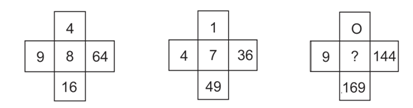a) 10

b) 01

c) 02

d) 16

4) A child starts walking from point ‘P’ and then reach to point ‘Q’ and angle between P & Q is 60o than how many distances have left is the child cover the remaining distance that is opposite directions of the child (i.e. the angle between P & Q is 3000)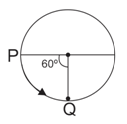a) 5πr / 3

b) 2πr / 5

c) 2πr / 3

d) 3πr / 2

5) A clock is started at 7 o’ clock in the morning. Through how many degrees will the hour hand rotate when the clock shows 11 o’clock?

a) 900

b) 1000

c) 1100

d)1200

6) From the given alternatives, select the word which cannot be formed giving the letters of the given word ‘SUSTAINABLE’.

a) ABLE

b) SUSTAIN

c) NOBLE

d) SUTABLE

7) Pointing to a woman is the photo-frame, Aadil said, “the only daughter of her grandfather is my wife”. How is Aadil related to that woman?

a) Brother

b) Uncle

c) Father

d) Maternal Uncle

8) What is the next letter in the series?

B, E, I, N, ?

a) S

b) T

c) O

d) L

9) If in certain language, “741” means “My favorite menu”. “264” means “Your favorite food” and “4125” means “All your favorite menu”, then what is code for “My Food”?

a) 17

b) 56

c) 72

d) 67

10) If in certain language, “741” means “My favorite menu”. “264” means “Your favorite food” and “4125” means “All your favorite menu”, then what is code for “All Recipe”?

a) 26

b) 15

c) 53

d) 43

11 => 112 + 11 = 121 + 11 = 132

13 => 132 + 13 = 169 + 13 = 182

8 => 82 + 8 = 64 + 8 = 72

14 => 142 + 14 = 196 + 14 = 210≠212

17 => 17 – 1 = 16 × 4 = 647

15 => 15 – 1 = 14 × 4 = 565

13 => 13 – 1 = 12 × 4 = 483

19 => 19 – 1 = 18 × 4 = 727 ≠ 721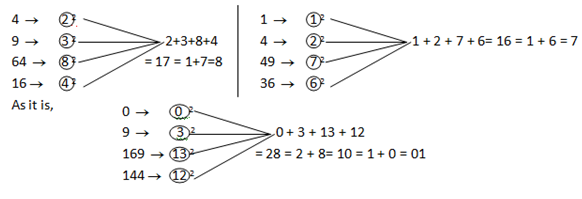Distance between P & Q at an angle of 600: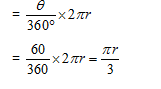So, the remaining distance between P & Q at an angle of 300o in the opposite direction.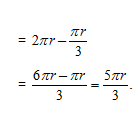Angle traced by hour hand in 12 h = 360o

Angle traced by hour hand is 4 h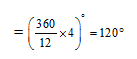‘NOBLE’ is the right option because ‘O’ of ‘NOBLE’ is absent in the given word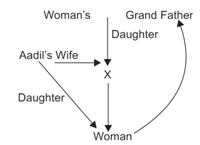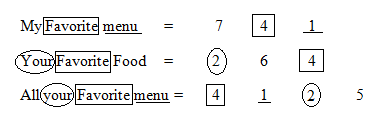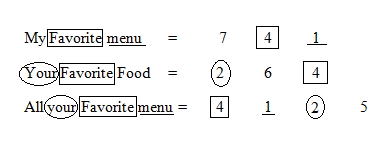*********************************